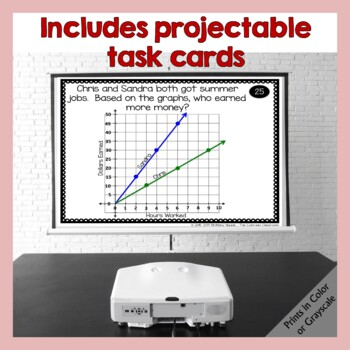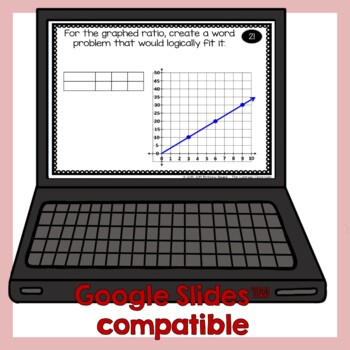# Graphing Ratios Task Cards with Mini LessonSubject
Resource Type
File Type

PDF

(19 MB|66 pages)
Standards
Also included in:
1. This bundle assembles three great ratio task card lessons together for one incredible price. Have students learn about ratios and equivalent ratios, understand unit rates and how to find them, and graph ratios on a coordinate system. Each task card set includes a mini-lesson of 12-15 pages in leng
\$12.50
\$7.60
Save \$4.90
• Product Description
• StandardsNEW

Do you want your students to be able to name coordinates on a grid and find similar ratios? What about graphing ratios using word problems? If so, then this resource is right up your alley. It includes 32 graphing ratio task cards (4 to a page) plus a projectable 13-page mini lesson to go with them. The mini-lesson includes graphing rates, finding similar ratios, and comparing and analyzing ratios.

• name coordinates on a grid
• identify missing coordinates in a series
• find a similar ratio that can be graphed on the same line
• complete a table using a graphed ratio
• graph a ratio using a word problem
• create their own word problem to fit a graphed ratio
• compare and analyze two ratios and answer a question

Now it also includes projectable task cards so that the class can get started together, you can complete it as class, or you can review ones where students get stuck.

This is the third set of task cards in a set on ratios. To get the others, or a bundle of all of them, please see here.

Ratios & Equivalent Ratios

You may also like

Whole Number Review Scavenger Hunt

Mean, Median, Mode, and Range Exploration Activity

Order of Operations Activity

Customer Tips:

How to get TpT credit to use on future purchases:

* Please go to your My Purchases page (you may need to login. Beside each purchase you'll see a Provide Feedback button. Simply click it and you will be taken to a page where you can give a quick rating and leave a short comment for the product. Each time you give feedback, TpT gives you feedback credits that you use to lower the cost of your future purchases. I value your feedback greatly as it helps me determine which products are most valuable to you and for your classroom, so I can create more of what you're looking for. :)

Become a Follower:

* Look for the green star next to my store logo and click it to become a follower.

* Be the first to know about my new discounts, freebies, and product launches.

Identify the constant of proportionality (unit rate) in tables, graphs, equations, diagrams, and verbal descriptions of proportional relationships.
Decide whether two quantities are in a proportional relationship, e.g., by testing for equivalent ratios in a table or graphing on a coordinate plane and observing whether the graph is a straight line through the origin.
Recognize and represent proportional relationships between quantities.
Make tables of equivalent ratios relating quantities with whole-number measurements, find missing values in the tables, and plot the pairs of values on the coordinate plane. Use tables to compare ratios.
Use ratio and rate reasoning to solve real-world and mathematical problems, e.g., by reasoning about tables of equivalent ratios, tape diagrams, double number line diagrams, or equations.
Total Pages
66 pages
Included
Teaching Duration
50 minutes
Report this Resource to TpT
Reported resources will be reviewed by our team. Report this resource to let us know if this resource violates TpT’s content guidelines.\$3.60
List Price:
\$4.50
You Save:
\$0.90